# Thin Arc Clamped in Plane BendingThis test lets you validate the combined case. The aim is to compare a static case (where several forces are applied) and a combination of static cases (the set of forces of each static case is therefore equivalent to the one of the first configuration).Reference:

• AFNOR technique, SFM Paris, Guide de validation des progiciels de calcul de structures, SSLL 06/89, p30, 1990.

• Results of the first configuration (all forces applied are located in one static case)

##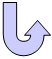Specifications

### Geometry Specifications

 r = 3 m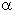= 90°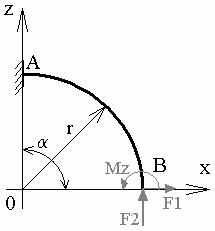Hollow circular section:  rext = 0.01 m  rint = 0.008 m

### Analysis Specifications

 Young Modulus (material): E = 200 GPa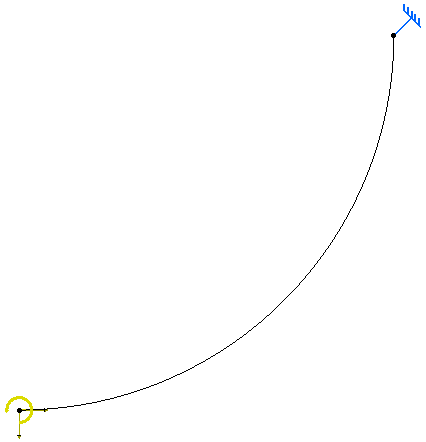Mesh Specifications: Linear beam elements Mesh size: 50 mm Restraints:Clamp on A Loads: At point B: Distributed force defined in the first static case: F1x = 10 N Distributed force defined in the second static case: F2y = 5 N Moment defined on the third static case: Mz = 8 N.m For the combined case, the three static cases have the same coefficient: 1

##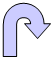Results

### Analytical Solution

• At point B: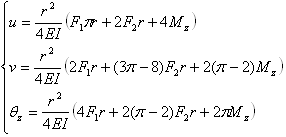Sensors Values Translation displacements uB (mm) 379.1 Translation displacements vB (mm) 241.7 Rotation displacements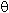zB (rad) 0.1654

### Computed Results

• Case computed with only one static case, gathering all applied forces: Sensors Values uB (mm) 378.97885 vB (mm) 241.73313zB (rad) 0.165
• Case computed with the combined case:

 Sensors Analytic reference values One static case reference values Combined case values uB (mm) 379.1 378.97885 378.97888 vB (mm) 241.7 241.73313 241.73313zB (rad) 0.1654 0.165 0.165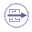To Perform the Test:

The thin_arc_combined.CATAnalysis document presents a complete analysis of this case.

The thin_arc_total.CATAnalysis document presents a complete analysis of this case.

To compute the case, proceed as follow:

1. Open one of the CATAnalysis document.

2. Compute the case in the Generative Structural Analysis workbench.

3. Create local sensors (Displacement vectors and Rotation vector).